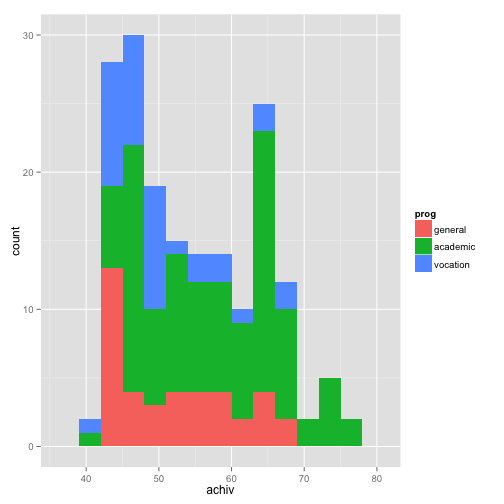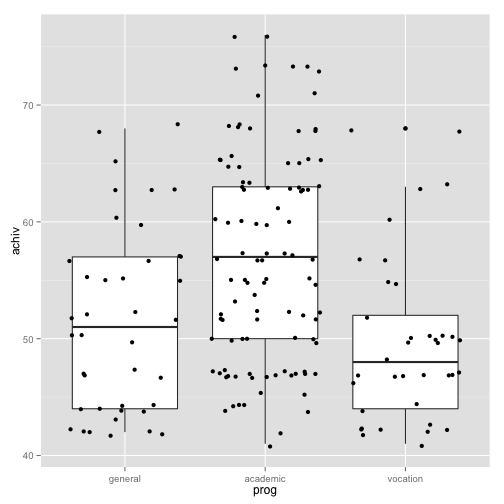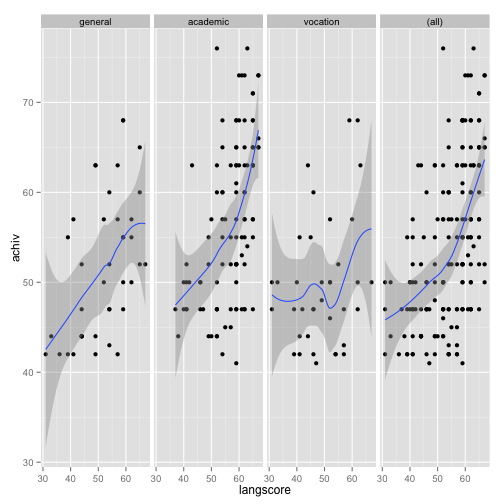# Truncated regression

Truncated regression is used to model dependent variables for which some of the observations are not included in the analysis because of the value of the dependent variable.

```require(foreign)
library(truncreg)
```
```## Loading required package: maxLik
##
## Please cite the 'maxLik' package as:
## Henningsen, Arne and Toomet, Ott (2011). maxLik: A package for maximum likelihood estimation in R. Computational Statistics 26(3), 443-458. DOI 10.1007/s00180-010-0217-1.
##
## If you have questions, suggestions, or comments regarding the 'maxLik' package, please use a forum or 'tracker' at maxLik's R-Forge site:
## https://r-forge.r-project.org/projects/maxlik/
```
```library(boot)
library(ggplot2)
```

## Examples of truncated analysis

• Example 1. A study of students in a special GATE (gifted and talented education) program wishes to model achievement as a function of language skills and the type of program in which the student is currently enrolled. A major concern is that students are required to have a minimum achievement score of 40 to enter the special program. Thus, the sample is truncated at an achievement score of 40.

• Example 2. A researcher has data for a sample of Americans whose income is above the poverty line. Hence, the lower part of the distribution of income is truncated. If the researcher had a sample of Americans whose income was at or below the poverty line, then the upper part of the income distribution would be truncated. In other words, truncation is a result of sampling only part of the distribution of the outcome variable.

# Gifted and talented education data

Let's pursue Example 1 from above. We have a hypothetical data file, `truncreg.dta`, with 178 observations. The outcome variable is called `achiv`, and the language test score variable is called `langscore`. The variable `prog` is a categorical predictor variable with three levels indicating the type of program in which the students were enrolled. Let's look at the data. It is always a good idea to start with descriptive statistics.

```dat <- read.dta("http://www.karlin.mff.cuni.cz/~pesta/prednasky/NMFM404/Data/truncreg.dta")

summary(dat)
```
```##        id             achiv         langscore           prog
##  Min.   :  3.00   Min.   :41.00   Min.   :31.00   general : 40
##  1st Qu.: 55.25   1st Qu.:47.00   1st Qu.:47.50   academic:101
##  Median :102.50   Median :52.00   Median :56.00   vocation: 37
##  Mean   :103.62   Mean   :54.24   Mean   :54.01
##  3rd Qu.:151.75   3rd Qu.:63.00   3rd Qu.:61.75
##  Max.   :200.00   Max.   :76.00   Max.   :67.00
```

```# histogram of achiv coloured by program type
ggplot(dat, aes(achiv, fill = prog)) + geom_histogram(binwidth = 3)
``````# boxplot of achiv by program type
ggplot(dat, aes(prog, achiv)) + geom_boxplot() + geom_jitter()
``````ggplot(dat, aes(x = langscore, y = achiv)) +
geom_point() +
stat_smooth(method = "loess") +
facet_grid(. ~ prog, margins=TRUE)
```## Analysis methods you might consider

Below is a list of some analysis methods you may have encountered. Some of the methods listed are quite reasonable, while others have either fallen out of favor or have limitations.

• OLS regression - You could analyze these data using OLS regression. OLS regression will not adjust the estimates of the coefficients to take into account the effect of truncating the sample at 40, and the coefficients may be severely biased. This can be conceptualized as a model specification error (Heckman, 1979).
• Truncated regression - Truncated regression addresses the bias introduced when using OLS regression with truncated data. Note that with truncated regression, the variance of the outcome variable is reduced compared to the distribution that is not truncated. Also, if the lower part of the distribution is truncated, then the mean of the truncated variable will be greater than the mean from the untruncated variable; if the truncation is from above, the mean of the truncated variable will be less than the untruncated variable.
• These types of models can also be conceptualized as Heckman selection models, which are used to correct for sampling selection bias.
• Censored regression - Sometimes the concepts of truncation and censoring are confused. With censored data we have all of the observations, but we don't know the "true" values of some of them. With truncation, some of the observations are not included in the analysis because of the value of the outcome variable. It would be inappropriate to analyze the data in our example using a censored regression model.

# Truncated regression modeling

Below we use the `truncreg` function in the `truncreg` package to estimate a truncated regression model. The `point` argument indicates where the data are truncated, and the direction indicates whether it is left or right truncated.

```m <- truncreg(achiv ~ langscore + prog, data = dat, point = 40, direction = "left")

summary(m)
```
```##
## Call:
## truncreg(formula = achiv ~ langscore + prog, data = dat, point = 40,
##     direction = "left")
##
##
## Coefficients :
##              Estimate Std. Error t-value  Pr(>|t|)
## (Intercept)  11.29942    6.77173  1.6686   0.09519 .
## langscore     0.71267    0.11446  6.2264 4.773e-10 ***
## progacademic  4.06267    2.05432  1.9776   0.04797 *
## progvocation -1.14422    2.66958 -0.4286   0.66821
## sigma         8.75368    0.66647 13.1343 < 2.2e-16 ***
## ---
## Signif. codes:  0 '***' 0.001 '**' 0.01 '*' 0.05 '.' 0.1 ' ' 1
##
## Log-Likelihood: -591.31 on 5 Df
```

• In the table of coefficients, we have the truncated regression coefficients, the standard error of the coefficients, the Wald z-tests (coefficient/se), and the p-value associated with each z-test (shown as t-values).
• The ancillary statistic/sigma is equivalent to the standard error of estimate in OLS regression. The value of 8.76 can be compared to the standard deviation of achievement which was 8.96. This shows a modest reduction. The output also contains an estimate of the standard error of sigma.
• The variable `langscore` is statistically significant. A unit increase in language score leads to a .71 increase in predicted achievement. One of the indicator variables for `prog` is also statistically significant. Compared to general programs, academic programs are about 4.07 higher. To determine if `prog` itself is statistically significant, we can test models with it in and out for the two degree-of-freedom test of this variable.
```# update old model dropping prog
m2 <- update(m, . ~ . - prog)

pchisq(-2 * (logLik(m2) - logLik(m)), df = 2, lower.tail = FALSE)
```
```## 'log Lik.' 0.02516651 (df=3)
```

The two degree-of-freedom chi-square test indicates that `prog` is a statistically significant predictor of `achiv`. We can get the expected means for each program at the mean of `langscore` by reparameterizing the model.

```# create mean centered langscore to use later
dat <- within(dat, {
mlangscore <- langscore - mean(langscore)
})

malt <- truncreg(achiv ~ 0 + mlangscore + prog, data = dat, point = 40)
summary(malt)
```
```##
## Call:
## truncreg(formula = achiv ~ 0 + mlangscore + prog, data = dat,
##     point = 40)
##
##
## Coefficients :
##              Estimate Std. Error t-value  Pr(>|t|)
## mlangscore    0.71259    0.11448  6.2248  4.82e-10 ***
## proggeneral  49.78926    1.89714 26.2443 < 2.2e-16 ***
## progacademic 53.85340    1.15012 46.8242 < 2.2e-16 ***
## progvocation 48.65315    2.14049 22.7299 < 2.2e-16 ***
## sigma         8.75545    0.66684 13.1299 < 2.2e-16 ***
## ---
## Signif. codes:  0 '***' 0.001 '**' 0.01 '*' 0.05 '.' 0.1 ' ' 1
##
## Log-Likelihood: -591.31 on 5 Df
```

Notice all that has changed is the intercept is gone and the program scores are now the expected values when `langscore` is at its mean for each type of program.

We could also calculate the bootstrapped confidence intervals if we wanted to. First, we define a function that returns the parameters of interest, and then use the `boot` function to run the bootstrap.

```f <- function(data, i) {
require(truncreg)
m <- truncreg(formula = achiv ~ langscore + prog, data = data[i, ], point = 40)
as.vector(t(summary(m)\$coef[, 1:2]))
}

set.seed(10)

(res <- boot(dat, f, R = 1200, parallel = "snow", ncpus = 4))
```
```##
## ORDINARY NONPARAMETRIC BOOTSTRAP
##
##
## Call:
## boot(data = dat, statistic = f, R = 1200, parallel = "snow",
##     ncpus = 4)
##
##
## Bootstrap Statistics :
##        original        bias    std. error
## t1*  11.2994158  0.3039135945  5.93277633
## t2*   6.7717257 -0.0559320949  0.86199032
## t3*   0.7126732 -0.0040554268  0.09651639
## t4*   0.1144602 -0.0006848377  0.01372122
## t5*   4.0626698 -0.0536791573  2.03318632
## t6*   2.0543191 -0.0014251852  0.24110408
## t7*  -1.1442162  0.0213603619  2.87208656
## t8*   2.6695799  0.0121405706  0.29443074
## t9*   8.7536778 -0.1094948867  0.54996087
## t10*  0.6664744 -0.0109213295  0.07535149
```

We could use the bootstrapped standard error to get a normal approximation for a significance test and confidence intervals for every parameter. However, instead we will get the percentile and bias adjusted 95 percent confidence intervals, using the `boot.ci` function.

```# basic parameter estimates with percentile and bias adjusted CIs
parms <- t(sapply(c(1, 3, 5, 7, 9), function(i) {
out <- boot.ci(res, index = c(i, i + 1), type = c("perc", "bca"))
with(out, c(Est = t0, pLL = percent, pUL = percent, bcaLL = bca,
bcaLL = bca))
}))

# add row names
row.names(parms) <- names(coef(m))
# print results
parms
```
```##                     Est         pLL        pUL       bcaLL      bcaLL
## (Intercept)  11.2994158 -1.25801473 22.2970167 -3.72311743 21.3202749
## langscore     0.7126732  0.53860641  0.9159501  0.55079089  0.9435087
## progacademic  4.0626698  0.05802952  8.0112185  0.08421688  8.0425925
## progvocation -1.1442162 -6.80545872  4.2770202 -6.84361734  4.2502140
## sigma         8.7536778  7.67372151  9.7919028  7.88960453 10.1104743
```

The conclusions are the same as from the default model tests. You can compute a rough estimate of the degree of association for the overall model, by correlating `achiv` with the predicted value and squaring the result.

```dat\$yhat <- fitted(m)

# correlation
(r <- with(dat, cor(achiv, yhat)))
```
```##  0.5524392
```
```# rough variance accounted for
r^2
```
```##  0.305189
```

The calculated value of .31 is rough estimate of the R2 you would find in an OLS regression. The squared correlation between the observed and predicted academic aptitude values is about 0.31, indicating that these predictors accounted for over 30% of the variability in the outcome variable.

## Things to consider

• The `truncreg` function is designed to work when the truncation is on the outcome variable in the model. It is possible to have samples that are truncated based on one or more predictors. For example, modeling college GPA as a function of high school GPA (HSGPA) and SAT scores involves a sample that is truncated based on the predictors, i.e., only students with higher HSGPA and SAT scores are admitted into the college.
• You need to be careful about what value is used as the truncation value, because it affects the estimation of the coefficients and standard errors. In the example above, if we had used `point` = 39 instead of `point` = 40, the results would have been slightly different. It does not matter that there were no values of 40 in our sample.

## References

• UCLA: IDRE (Institute for Digital Research and Education). Data Analysis Examples. from http://www.ats.ucla.edu/stat/dae/ (accessed January 31, 2014)
• Long, J. S. (1997). Regression Models for Categorical and Limited Dependent Variables. Thousand Oaks, CA: Sage Publications.
• Greene, W. H. (2003). Econometric Analysis, Fifth Edition. Upper Saddle River, NJ: Prentice Hall.
• Heckman, J. J. (1979). Sample selection bias as a specification error. Econometrica, Volume 47, Number 1, pages 153 - 161.